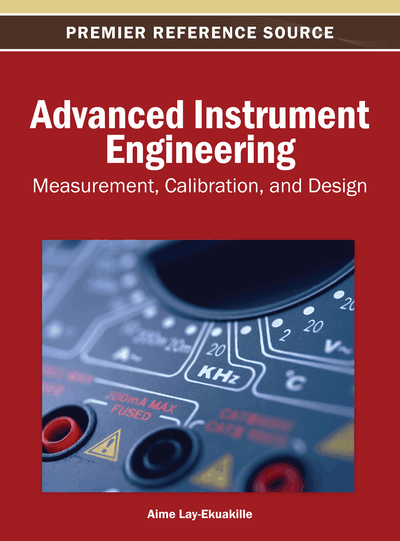# An Observation on Least Action Principle in Classical Mechanics Oriented on the Evaluation of Relativistic Error Concerning the Measurements of Light Propagating in a Liquid

Alessandro Massaro (Italian Institute of Technology, Italy) and Piero Adriano Massaro (University of Bari, Italy)
DOI: 10.4018/978-1-4666-4165-5.ch013

## Abstract

The authors prove that the standard least action principle implies a more general form of the same principle by which they can state generalized motion equation including the classical Euler equation as a particular case. This form is based on an observation regarding the last action principle about the limit case in the classical approach using symmetry violations. Furthermore the well known first integrals of the classical Euler equations become only approximate first integrals. The authors also prove a generalization of the fundamental lemma of the calculus of variation and we consider the application in electromagnetism.
Chapter Preview
Top

## 2. The Action Functional In Different Sets Of Admissible Functions

Let us consider the action functional for a single particle moving along x-axis

(1) where L(t,x,is the Lagrangian. If x(t) is the real physical curve described by the particle (trajectory), such that x(t1)=a, x(t2)=b, we consider the functional (1) in the following sets of admissible functions

(2)

Here D1(t1,t2) is the space of all functions defined on the interval [t1,t2] which are continuous and have continuous first derivatives; h(t) is an arbitrary variable function, h0(t) and h1(t) are arbitrary but fixed functions; α is a real variable parameter. In Figure 1 is reported an example of x2-trajectory.

The increment of action functional corresponding to the increment h(t) of x(t) is written as

(3) where δS[x;h] is the differential of the functional and ε→0 for ||h||→0. The last action principle states that in the set Γ1 we have

(4)

We now observe that (δS(1)[x]=0)→ (δS(2)[x]=0)→ (δS(3)[x]=0).

In fact an explicit form of the Formula (4) can be derived by using the Taylor’s theorem. So we have

(5) where the subscript denotes partial derivatives with respect to the corresponding arguments, and the dots denote terms of order higher than 1 relative to h and## Complete Chapter List

Search this Book:
Reset